﻿ Numerical Simulation and Experiments of Barite Sag in Horizontal Annulus

### Numerical Simulation and Experiments of Barite Sag in Horizontal Annulus

Yahya Hashemian, Stefan Miska, Mengjiao Yu, Evren Ozbayoglu, Nicholas TakachOPEN ACCESSPEER-REVIEWED

## Numerical Simulation and Experiments of Barite Sag in Horizontal Annulus

Yahya Hashemian1, Stefan Miska1,, Mengjiao Yu1, Evren Ozbayoglu1, Nicholas Takach1

1Petroleum Enginerring Department, University of Tulsa, Tulsa, USA

### Abstract

Under certain drilling conditions, the weighting material particles such as barite can settle out of the drilling fluid. This phenomenon, known as barite sag, can lead to a number of drilling problems including lost circulation, well control difficulties, poor cement job, and stuck pipe. This study investigates barite sag, both experimentally and numerically, in the annulus under flow conditions. Experimental work has been conducted on a large flow loop to investigate the effects of major drilling parameters on barite sag by measuring the circulating fluid density. Results of the tests indicate that the highest sag occurs at low annular velocities and rotational speed and also at high inclination angles. It was observed that at inclination angles less than 60°, for any annular velocity, barite sag is not significant. Eccentricity of a non-rotating inner pipe did not have a significant effect on barite sag. However, effects of inner pipe rotation on barite sag for an eccentric annulus are more significant than concentric case. The simulation part of this study is based on a proposed particle tracking method called “Particle Elimination Technique”. The traveling path of each solid particle is assumed to be a function of size and shape of the particle, fluid velocity and rheology. Based on the estimated traveling path of particles, density of the fluid is updated considering the number of particles whose paths lead to the bottom of the annulus and become motionless. In order to capture the complexities associated with the solid-liquid flow, a lift force is assigned to the solid particles that enable adjustment of the model with experimental results. Comparing the results of numerical simulation to the experimental study on the effects of annular velocity on barite sag in a horizontal annulus shows a good agreement. The numerical simulation was modified from laboratory scale to real wellbore dimensions for practical drilling applications. Results of the simulation show prediction of the density of the drilling fluid in the horizontal section of a wellbore with various lengths and dimensions under different annular velocities.

### At a glance: Figures

123
Prev Next

• Hashemian, Yahya, et al. "Numerical Simulation and Experiments of Barite Sag in Horizontal Annulus." American Journal of Numerical Analysis 2.1 (2014): 14-19.
• Hashemian, Y. , Miska, S. , Yu, M. , Ozbayoglu, E. , & Takach, N. (2014). Numerical Simulation and Experiments of Barite Sag in Horizontal Annulus. American Journal of Numerical Analysis, 2(1), 14-19.
• Hashemian, Yahya, Stefan Miska, Mengjiao Yu, Evren Ozbayoglu, and Nicholas Takach. "Numerical Simulation and Experiments of Barite Sag in Horizontal Annulus." American Journal of Numerical Analysis 2, no. 1 (2014): 14-19.

 Import into BibTeX Import into EndNote Import into RefMan Import into RefWorks

### 1. Introduction

During conventional drilling operation, controlling the sub-surface pressure is achieved by use of high density drilling fluid, which contains high density minerals such as barite. However, under certain drilling conditions, the weighting material particles can settle out of the drilling fluid suspension. This phenomenon, known as barite sag, can lead to a number of drilling problems including lost circulation, well control difficulties, poor cement job, and stuck pipe. The term “barite sag” is used because barite is the most popular weighting material used in drilling fluids to increase density. Unfortunately, barite sag is proving to be a real question for drilling industry and the problem is often unanticipated. Although much has been done, we have no universal solutions that can explain and predict the phenomenon. Moreover, recent advances in drilling technology have resulted in greater numbers of directional wells which limit the application of common remedies to mitigate sag.

Some mathematical models are presented using the principles of continuum mechanics. They are focused on the period during which pumping and rotation are stopped (Acrivos et al. 1979 and Pasaly et al. 2007).

It has been shown that sag can be reduced by increasing pipe rotation (Bern et al. 1998). On the other hand it is pointed out that the rotation serves to generate a low shear rate environment in which barite particles could settle and slip downward more easily (Hemphill et al. 2006). These two conclusions contradict each other.

At high velocity, particularly when turbulence was observed, sag beds are removed and incorporated back into the mud (Hanson et al. 1990). At annular velocities above 100 ft/min, the “magnitude of dynamic sag” is small compared to lower annular velocities. The highest sag occurs at an annular velocity of 40 ft/min. (Dye et al. 1999).

Hanson et al. (1990) showed that the sag tendency is significantly higher in wells with inclination angles of 30-60° from vertical. Bern et al. (1998) concluded that significant barite sag was measured at angles as high as 75° and the most critical range is 60 to 75°.

Eccentricity of drill pipe is shown to exacerbate barite sag (Bern et al. 1998).

Nguyen (2009) conducted several experiments on annular and pipe flow loop. His results show that even at low annular velocities (16 ft/minute) sag can be mitigated with pipe rotation speeds of 20 RPM. He also indicated that pipe rotation has a greater impact on reducing sag when the pipe is eccentric than when it is concentric. His experiments showed that at low annular velocity (lower than 40 ft/min), a barite bed is formed very quickly at the lower side of the test section.

### 2. Mathematical Approach

Simulation part of study is based on a proposed particle tracking method called “Particle Elimination Technique”. An effort is done to simulate the flow of drilling fluid in the annulus. Flow of a non-Newtonian fluid in an annulus is solved numerically to find its velocity profile. Falling velocity of a single particle in power law fluid is assigned to the particles. Based on the diameter of each particle and its location in the annulus, its traveling path is estimated and then particles whose traveling path leads to the bottom of the annulus are set to be stationary and then removed from the main flow stream of the fluid. Density of the fluid is updated, taking into account the portion of particles which are set to stationary.

Lift force due to irregular shape of barite particles is assigned to each particle and the resultant force is updated for calculation of the falling velocity.

Simulation of barite sag in this work is divided into two parts: 1) modeling of laminar flow of non-Newtonian fluid in annulus to obtain velocity profile; and 2) consideration of the solid particles in the fluid to predict the particle traveling path and time.

### 3. First Part of Mathematical Approach: Obtain Velocity Profile of Laminar Flow of Non-Newtonian Fluid in Eccentric Annulus

To simulate fluid flow in an eccentric annulus when the drill pipe is stationary, governing equations for flow in an eccentric annulus are developed using a Cartesian coordinate system. The Yield Power Law model is used to represent the rheology of the fluid. To solve the flow equations, a boundary fitted coordinate system is utilized to apply numerical technique to the equations. Geometry as well as equations are transformed into a computational domain in a boundary-fitted coordinate system (Figure 1). Equations are discredited and an iterative over relaxation method is used to solve for the velocity field at each grid point, knowing frictional pressure loss in axial direction of the well bore. A relaxation technique is used (after Azouz 1994) to speed up convergence of the solution.

For axial laminar flow, the equation of motion will take the form: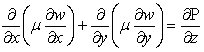(1)

Where, w is the fluid velocity in axial direction and μ is the viscosity that is a function of shear rate. The viscosity function depends on the rheology of the fluid. The shear rate for axial flow can be expressed as: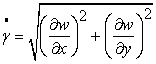(2)

The applied boundary conditions are zero velocity on the walls of the inner pipe and outer pipe.

Unlike Newtonian fluids, a non-Newtonian fluid does not have a constant viscosity. However, in numerical modeling, the concept of viscosity is used for non-Newtonian fluids to make the governing equations similar to Newtonian fluids. This viscosity is known as apparent viscosity that is a function of shear rate and depends on the rheology of the fluid. Apparent viscosity is generally defined as: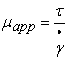(3)

For YPL fluids, the apparent viscosity will be: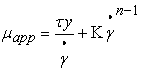(4)
Figure 1. Grid network in boundary-fitted coordinate (two top figures) and velocity profile in 3-D format for annular flow of a Newtonian and non-Newtonian fluid in eccentric annulus (two bottom Figures)

The result of this part of the simulation is a velocity profile in the annulus as shown in Figure 1 (bottom). Having the velocity field, flow rate is calculated through numerical integration over the flow region.

### 4. Comparison of the Results of the First Part of Simulation with Experiments

Results of the simulation were compared with experimental work conducted at The University of Tulsa drilling research projects (TUDRP) by Ahmed (2005).

Figure 2. Dynamic testing facility by Ramadan Ahmed, 2005 (Top) and comparison with the numerical simulation of this study (Bottom)

In his work, extensive flow experiments with polymer-based fluids were carried out using a flow loop (Figure 2). Different formulations of test fluids were prepared. Eccentricities of the annular test sections were varied from 0% to 100%. Annular test sections with four different diameter ratios were investigated. Flow rate was varied from 0.024 to 21.91 gal/min [0.09 to 82.82 liter/min].

Figure 3. Dimensionless velocity profile of power law fluid in concentric annulus based on the numerical simulation (Left) and Velocity components of a solid particle in concentric annulus

Figure 3 (left) shows the results of the simulation on predicting the velocity profile for a wide range of power law fluid behavior index values of 0.1 to 1.0 (Newtonian). In this figure, the dimensionless radius is the ratio of inner pipe radius to outer pipe radius. It starts with 0.3 (on the outer wall of drill pie) and ends with 1.0 (on the inner wall of casing). The calculated velocity is assumed to be equal to the axial velocity of the solid particles. The second component of the solid particle velocity is assumed to be in the direction of gravity as shown in Figure 3 (right).

4.1. Second Part of Mathematical Approach: Particle Elimination Technique

The second part of the simulation is based on a proposed particle tracking method called “Particle Elimination Technique”. At this technique, solid particles (barite) are taken into consideration. Each particle at one grid point is assumed to have two components of velocity. One component is in the direction of the axial fluid velocity with a magnitude of the fluid velocity calculated at that point. This component of the velocity is already calculated from numerical simulation in the first part of the simulation. The second component of velocity is assumed to be in the direction of gravity normal to the first component.

Considering a specific length of the annulus, and based on the diameter of each particle and its location in the annulus, traveling path is estimated (Figure 4). Particles whose traveling path leads to the bottom of the annulus are set as stationary and then removed from the main flow stream of the fluid. Density of the fluid is updated, taking into account the number of particles which are set as stationary. It is further assumed that if a particle can reach the bottom of the annulus (casing wall) during a specific time period, then all other particles below it, with the same size and density, have already reached the bottom during that time period.

Therefore, by calculating the location of one specific particle that can reach the lower wall of the annulus during a specific time period or length, we can predict settlement of all other particles below that particle. Thus, we can remove those particles from the flow and calculate a new concentration and density for the fluid.

4.2. Falling Velocity of a Single Particle in Power Law Fluid

In this study we used an experimental correlation proposed by Shah et al. (2007) for calculating the falling velocity of solid particles in a power law fluid in SI units: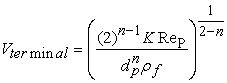(5)

Where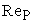is given by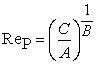and A, B and C could be found as: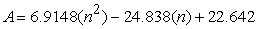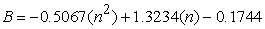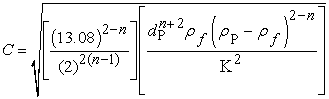4.3. Fluid Rheology

The drilling fluid used in this study is an oil based drilling fluid. Result of rheology test on this fluid is shown in Figure 5 (top).

Figure 5. Results of rheology test of the drilling fluid used in this study (top) and particle size distribution analysis on barite particles (bottom)

Assuming the average wall shear rate to be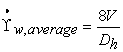and replacing the velocity with the lowest and the highest annular velocity in annulus, we have：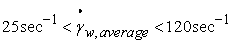.Conductinga rheology test on the drilling fluid shows that it has a good fit with the Power Law model in the range of the average shear rate shown in Figure 5 (top). At this point constants n and K of the Power Law model are obtained, which are needed for calculations of particle settling velocity. At the next stage we need to find the size associated with barite particles.

Barite used in this study complies with the API barite which has a wide range of diameter size and therefore needs to be carefully included in model. Result of particle size distribution test which was conducted by Baker Hughes Company is shown in Figure 5 (bottom).

4.4. Lift Force

It was observed from the experiments that barite sag decreases as annular velocity increases. Also it was observed that beyond a certain value of annular velocity, there is almost no barite sag. To capture this effect in the model, a lift force is assigned to the particles. The magnitude of the lift force was chosen so that the results of the simulation could match with results of experiments. The lift coefficient is a number that aerodynamicists use to calculate lift force in order to model the complex dependencies of the shape of solid bodies as well as some flow conditions on the lift. The lift coefficient, CL, is obtained as shown in Eq. 6: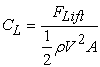(6)

where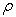is density, V is velocity and A is the cross-sectional area. In this study, the lift coefficient is adjusted based on the results of experimental studies on barite sag.

Lift force will reduce the normal weight of the particle, similar to buoyancy force. So the lift force can be incorporated into resultant density of particle as: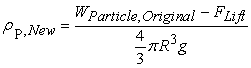(7)

### 5. Experimental Work

A large indoor flow loop was used for investigating the major drilling parameters affecting barite sag. It consists of a 35-ft long annulus with dimensions 4” x 2”. It has a 50-gal mud tank equipped with an agitator. A centrifugal pump circulates drilling fluid trough the annular test section and then returns it to the mud tank through a return pie. The inclination angle of the test section is varied from horizontal to vertical. Inner pipe can be rotated and its eccentricity can be set to concentric and fully eccentric positions. Flow rate and temperature of the flow is controlled accurately. Density of the fluid is measured and recorded continuously with time, using coriolis densitometers at both the inlet and outlet of the annular test section.

### 6. Results of Experimental Work

6.1. Effect of Inclination Angel, Rotation and Eccentricity on Barite Sag in Annulus

Results of experimental studies on the effects of inclination angle on barite sag for an eccentric annulus with a constant annular velocity are shown in Figure 7 (top). These plots show that barite sag decreases as inclination angle decreases. For inclination angles less than 60°, for a low annular velocity, barite sag is not significant.

Figure 7. Density reduction versus time in eccentric annulus for different inclination angles (top) and density reduction versus time in eccentric and concentric annulus with an without rotation (bottom)

Figure 7 (bottom) shows the effects of rotational speed on barite sag in eccentric and concentric annuli in a horizontal configuration. Comparing the concentric configuration versus the eccentric case shows that effect of rotational speed on barite sag reduction is more significant in eccentric case. The reason could be due to mechanical cleaning of the settled barite by the rotational movement of the inner pipe.

### 7. Comparison of Mathematical Modeling with Experiments

As shown in Figure 8, a comparison between the results of mathematical modeling and experimental studies of barite sag in a horizontal annulus for the tested velocities show generally good agreement.

Figure 8. Comparison of the results of simulation (thin lines) and experiments (thick lines) on the effects of annular velocity on barite sag for a horizontal concentric annulus

The Figure also shows that increase of the annular velocity can decrease barite sag significantly. However equilibrium in density change was not observed, unlike the results of decreasing inclination (Figure 7. top).

### 8. Application of Model in Wellbore Conditions

The numerical simulation was modified from laboratory scale to real wellbore dimensions for practical drilling applications. The main differences in modified model are the length of the well bore and the “infinite” mud tank volume. The annulus diameters as well as mud properties are chosen to be the same as the experimental configuration so that a comparison could be achieved. The assigned values to the length of the horizontal section of the wellbore are varied from small values (1,000 ft) to very large values (10,000 ft).

Figure 9. Results of simulation on prediction of barite sag at the end of horizontal section of well bore (kick off point) vs. time

The dotted lines in Figure 9 (bottom) show the average traveling time, which is calculated by dividing the length of the horizontal section by the average annular velocity. The above predictions show that barite sag is negligible after the drilling fluid reaches the end of the horizontal section (kick off point). This is in contrast with the results from experiments (as expected). The reason can be due to the difference in the volume of mud tank for the two cases under consideration. The mud tank volume for the lab configuration is too small compared to real field conditions.

### 9. Summary and Remarks

Results of the tests indicate that the highest sag occurs at low annular velocities and rotational speed and also at high inclination angles.

As annular velocity increases, barite sag decreases significantly.

It was observed that as inclination angle decreases, barite sag decreases consistently, which is in contradiction with some of the results in the literature. At inclination angles less than 60°, under any velocities, barite sag is not significant.

Eccentricity of a non-rotating inner pipe did not have a significant effect on barite sag. However, effects of inner pipe rotation on barite sag for an eccentric annulus are more significant than concentric case.

Comparing the results of numerical simulation to the experimental study on the effects of annular velocity on barite sag in a horizontal annulus shows a good agreement.

The numerical simulation was modified from laboratory scale to real wellbore dimensions for practical drilling applications. Results of the simulation allow prediction of the density of the drilling fluid in the horizontal section of a wellbore of various lengths and dimensions under different annular velocities.

### Acknowledgment

The author would like to thank the drilling research group at The University of Tulsa Drilling Research Projects (TUDRP) for its financial support of this study. TUDRP faculty members, including Dr. Stefan Miska and Dr. Mengjiao Yu, my advisor and co-advisor, respectively, as well as Dr. Evren Ozbayoglu and Dr. Tan Nguyenare also gratefully acknowledged for their advice and help. Thanks also goes to TUDRP research technicians, Mr. Randy Darden and Mr. Donald Harris for their help with the experimental setup. Appreciation is also expressed to Baker Hughes Company for providing the drilling fluid for our tests.

### References

  Acrivos, A. and Herbolzheimer, E. 1979. Enhanced Sedimentation in Settling Tanks with Inclined Walls. J. Fluid Mechanics, Vol. 92, part 3, 435-457.In article CrossRef  Ahmed, R. 2005. Experimental Study and Modeling of Yield Power-Law Fluid Flow in Pipes and Annuli. TUDRP Semi-Annual Report, May 2005.In article  Azouz, I. 1994. Numerical Simulation of Laminar and Turbulent Flows of Wellbore Fluids in Annular Passages of Arbitrary Cross-Section. University of Tulsa Drilling Research Projects, Ph.D. Dissertation.In article  Bern, P.A., van Oort, E., Neusstadt, B., Ebeltoft, H., Zurdo, C., Zamora, M., and Slater, K. 1998. Barite Sag: Measurement, Modelling and Management. IADC/SPE 47784, IADC/SPE Asia Pacific Drilling Tech, Jakarta, Indonesia, 7-9 Sept., and SPE Drilling & Completion, March 2000, 25-30.In article  Dye, W., Hemphill, T., Gusler, W., and Mullen, G. 1999. Correlation of Ultra-low Shear Rate Viscosity and Dynamic Barite Sag in Invert-Emulsion Drilling Fluids. SPE 56636, Houston, Texas, October.In article  Escudier, M.P, Oliveira, P.J., Pinho, F.T. 2001. Fully Developed Laminar Flow Of Purely Viscous Non-Newtonian Liquids Through Annuli, Including Of Eccentricity And Inner-Cylinder Rotation. Heat and Fluid Flow 23 (2001) 52-73.In article CrossRef  Haciislamoglu, M. 1989. Non-Newtonian Fluid Flow in Eccentric Annuli and Its Application to Petroleum Engineering Problems.In article  Hanson, P.M., Trigg, T.K., Rachal, G., and Zamora, M. 1990. Investigation of Barite “Sag” in Weighted Drilling Fluids in Highly Deviated Wells. SPE 20423, SPE ATCE, New Orleans, 23-26 Sept.In article  Hashemian A. Y. 2005. Numerical Simulation of Laminar Flow of Non-Newtonian Fluids in Eccentric Annuli. MSc. Thesis, University of Tulsa.In article  Hashemian, Yahya, 2012 “Prediction of Barite Sag in Horizontal Annular Flow,” SPE 160916-STU, International Student Paper Contest ATCE Texas.In article  Hashemian A. Y. 2012. Experimental Study and Modeling of Barite Sag in Annular Flow. PhDDissertation, University of Tulsa.In article  Hemphill, T., and Ravi, K. (2006) “Turning on Barite Sag with Drillpipe Rotation: Sometimes Surprises Are Really Not Surprises,” AADE, Fluids Conference, Houston, Texas, Nov.In article  Nguyen, T.C., Miska, S., Yu, M., and Takach, N., SPE, University of Tulsa (2009) “Predicting Dynamic barite Sag in Newtonian Oil Based Drilling Fluids” SPE 124137, SPE, New Orleans, 4-7 Oct.In article  Paslay, P.R., Sathuvalli, U.B., and Payne, M.L. (2007) “A Phenomenological Approach to Analysis of Barite Sag in Drilling Muds,” SPE 110404, SPE ATCE, Anaheim, 11-14 Nov.In article  Piercy, N.A.V., Hooper, M.S., Winny, H.F. 1933. Viscous flow through pipes with core. London Edinburgh Dublin Phil. Mag. J. Sci., 15 647-676.In article  Shah S.N., Fadili Y. El, Chhabra R.P. 2007. New model for single spherical particle settling velocity in power law (visco inelastic) fluids. Int. J. Multiphase Flow, 33 (2007), pp. 51-66.In article CrossRef